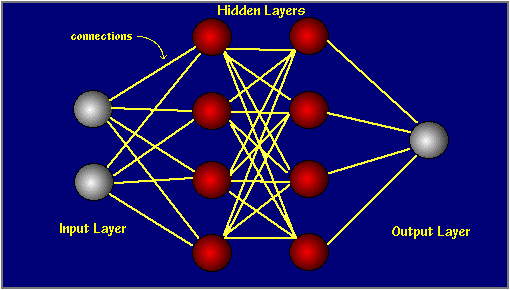# A Neural Network in 11 Lines of PythonArtificial Intelligence is getting embedded in our lives on a regular basis in form of various speech recognition software, digital assistants like Cortana or, the famous deep dreaming robot of Google – that turns pictures and video into horror movies. All this intelligence comes from a software called neural networks. Recently, a programmer shared a neural network tutorial from scratch starting from an 11 lines of neural network python code.

Artificial neural networks or ANNs are the processing devices that is modeled taking inspiration from the neuronal structure of a mammalian brain. A neural network is organized in layers that are made up of various nodes. The input layer communicated the input to other hidden layers that are connected to an output layer. There are many types of models used by neural networks. One of the most popular models is “backpropagation” – the backward propagation of errors.

Backpropagation is a common training method in neural networks that needs a known and desired output for calculating the losses/gradient. The programmer teaches a neural network implementation describing the inner workings of backpropagation via a very simple toy example.This backpropagation is shown via a small python implementation. Take a look at this neural network in 11 lines of python:

```X = np.array([ [0,0,1],[0,1,1],[1,0,1],[1,1,1] ])
y = np.array([[0,1,1,0]]).T
syn0 = 2*np.random.random((3,4)) - 1
syn1 = 2*np.random.random((4,1)) - 1
for j in xrange(60000):
l1 = 1/(1+np.exp(-(np.dot(X,syn0))))
l2 = 1/(1+np.exp(-(np.dot(l1,syn1))))
l2_delta = (y - l2)*(l2*(1-l2))
l1_delta = l2_delta.dot(syn1.T) * (l1 * (1-l1))
syn1 += l1.T.dot(l2_delta)
syn0 += X.T.dot(l1_delta)```This neural network attempts to use the input for predicting the output. Here programmer tries to predict the output column of the three input columns. Well, this was all I had to tell you about the neural network in 11 lines of python. This problem of simple backpropagation could be used to make a more advanced 2 layer neural network.

Visit this link to read further 2 and 3 layer neural network problems in python.

Try this 11 line python neural network and get more help on python in AI here.